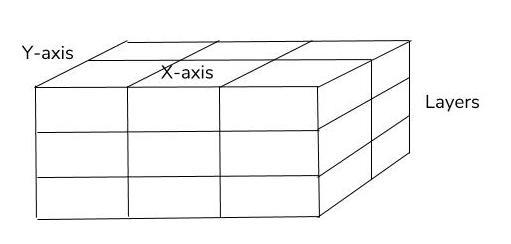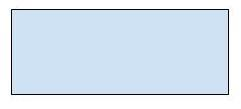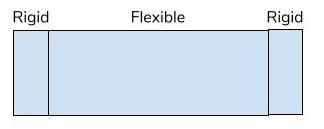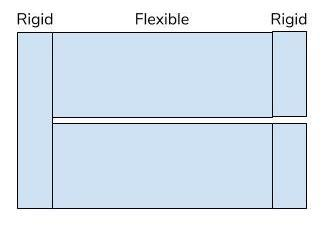# How to use Sections in CircuitData

The section part allows you to specify multiple sections. Used together with the “layers” it makes it possible to describe the product in three dimensions, like the illustration below shows:Picture a PCB seen from above:Most PCBs only have one section, like the illustration above. In CircuitData you could specify it like this (you have to describe at least one section):

```"sections": [
{
"name": "rigid1",
"in_x": ,
"in_y": ,
"mm2": 400
}
]
```

The “name” of it is up to you - you only use it to reference this section in the “layers” part of your CircuitData code.

Now picture a RigidFlex PCB from above (not a cross section):This PCB consists of three sections: two rigid parts and a flexible part. In the X-axis, it has three sections, in the Y-axis only one. You always start counting in the bottom left corner. In CircuitData, it would look something like this:

```"sections": [
{
"name": "rigid1",
"in_x": ,
"in_y": ,
"mm2": 100

},
{
"name": "flex1",
"in_x": ,
"in_y": ,
"mm2": 400
},
{
"name": "rigid2",
"in_x": ,
"in_y": ,
"mm2": 100
}
]
```

To make it even more complex, you could have a more RigidFlex with sections also in the Y-axis (still from above):This PCB consists of five sections: three rigid parts and two flexible parts. In the X-axis, it has three sections, in the Y-axis two. Again, remember that you always start counting the sections from the bottom left corner in both the X and the Y direction. In CircuitData, it would look something like this:

```"sections": [
{
"name": "rigid1",
"in_x": ,
"in_y": [1, 2],
"mm2": 200
},
{
"name": "flex1",
"in_x": ,
"in_y": ,
"mm2": 400
},
{
"name": "rigid2",
"in_x": ,
"in_y": ,
"mm2": 100
},
{
"name": "flex2",
"in_x": ,
"in_y": ,
"mm2": 400
},
{
"name": "rigid2",
"in_x": ,
"in_y": ,
"mm2": 100
},
]```

## In CircuitData

The structure in CircuitData places all sections in a “sections” array. In random order, a receiving system will/should parse all items in the array. Each item will have the following objects. Only name and mm2 are required:

• name

• Any text (string) to explain the section. This is used in layers to state which section the layers appears in (could be many)

• in_x

• An array of numbers (integers), could be only one, that states the sections position(s) in the X-axis.

• in_y

• An array of numbers (integers), could be only one, that states the sections position(s) in the Y-axis.

• size_x

• A number that states the size in millimeters in the X-axis

• size_y

• A number that states the size in millimeters in the Y-axis

• mm2

• A number that states the size of the section in square millimeters

Reply Oldest first
• Oldest first
• Newest first
• Active threads
• PopularLike Follow
• 1 yr agoLast active
• 276Views
• 1 Following